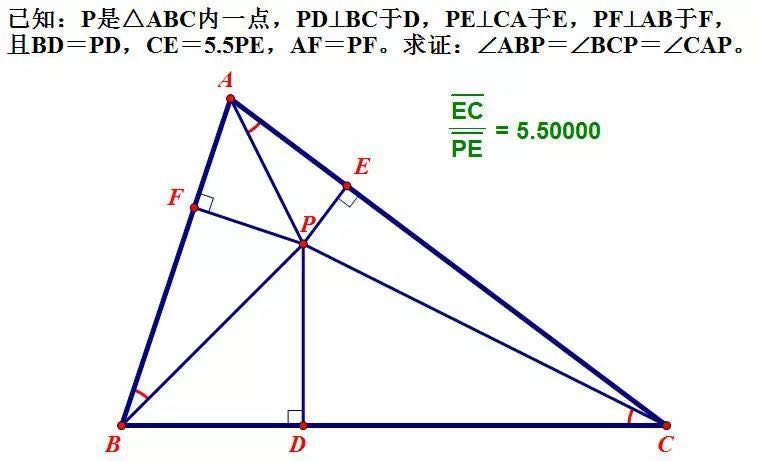###### back to index | new

The domain of the function $f(x) = \arcsin(\log_{m}(nx))$ is a closed interval of length $\frac{1}{2013}$ , where $m$ and $n$ are positive integers and $m>1$. Find the remainder when the smallest possible sum $m+n$ is divided by 1000.

In $\triangle ABC$, $\cos(2A-B)+\sin(A+B)=2$ and $AB=4$. What is $BC$?

If the circle $x^2 + y^2 = k^2$ covers at least one maximum and one minimal of the curve $f(x)=\sqrt{3}\sin\frac{\pi x}{k}$, find the range of $k$.

Let $x, y \in [-\frac{\pi}{4}, \frac{\pi}{4}], a \in \mathbb{Z}^+$, and $$\left\{ \begin{array}{rl} x^3 + \sin x - 2a &= 0 \\ 4y^3 +\frac{1}{2}\sin 2y +a &=0 \end{array} \right.$$ Compute the value of $\cos(x+2y)$

Let real numbers $x_1$ and $x_2$ satisfy $\frac{\pi}{2} > x_1 > x_2 > 0$, show $$\frac{\tan x_1}{x_1} > \frac{\tan x_2}{x_2}$$

As shown.Show that $|\sin(nx)|\le n|\sin(x)|$ for any positive integer $n$.

If $0 < \alpha < \beta < \frac{\pi}{2}$, show $$\frac{\cot\beta}{\cot\alpha}<\frac{\cos\beta}{\cos\alpha}<\frac{\beta}{\alpha}$$

In $\triangle{ABC}$ show that $\cos A +\cos B + \cos C \le\frac{3}{2}$.

Let $x\in(0, \pi/2)$ be expressed in radian. Explain why the relation $\sin x < x < \tan x$ hold?

Let $0 < x < \frac{\pi}{2}$. Show that $\sin x < x <\tan x$.